# 一个文件的开源项目，开启你的开源之旅OneFile 汇集了仅一个文件，好玩的开源项目。### 1、点击停球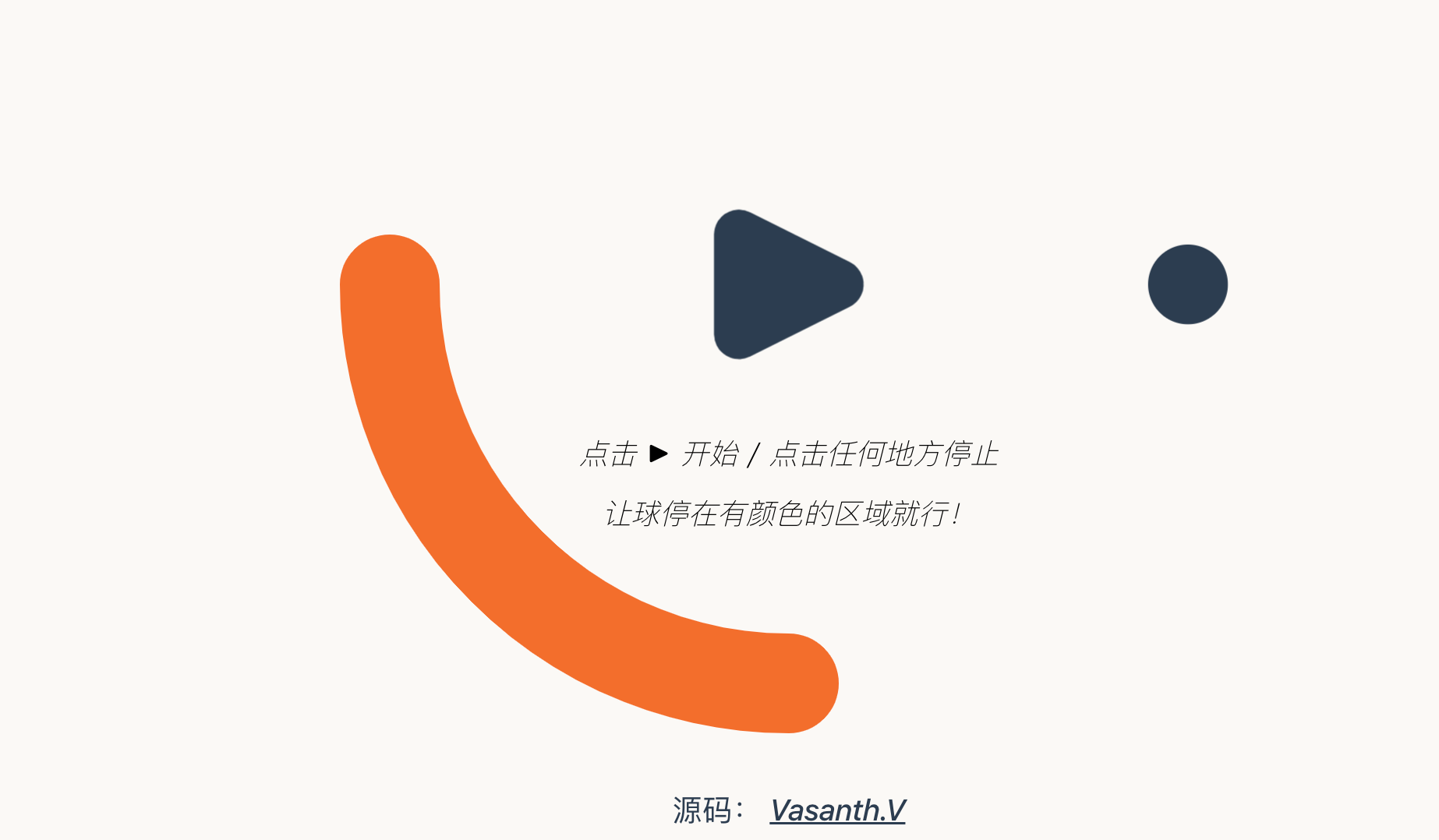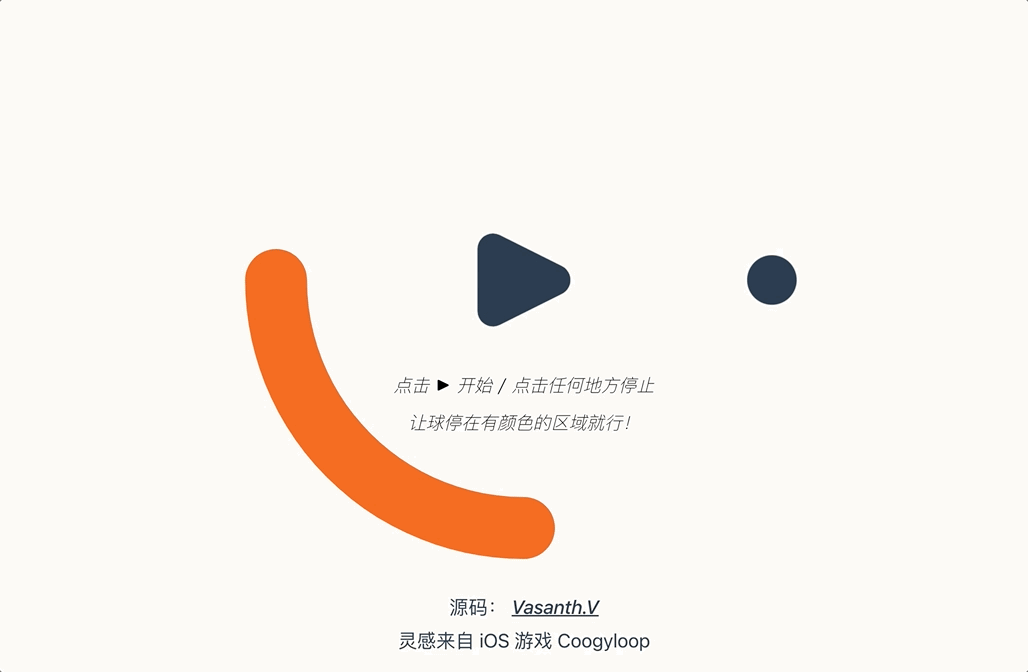### 2、番茄时钟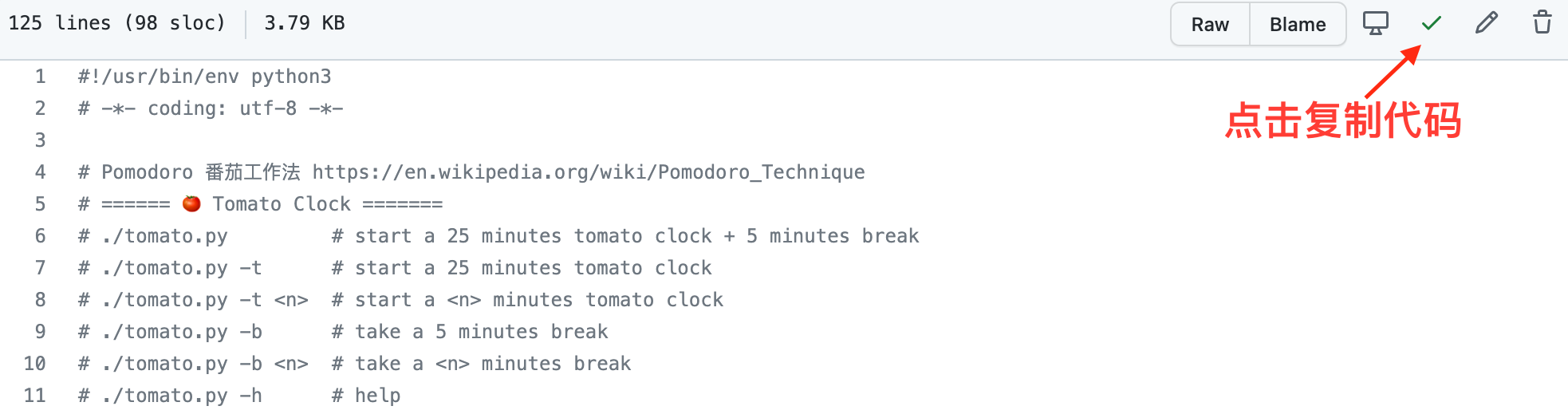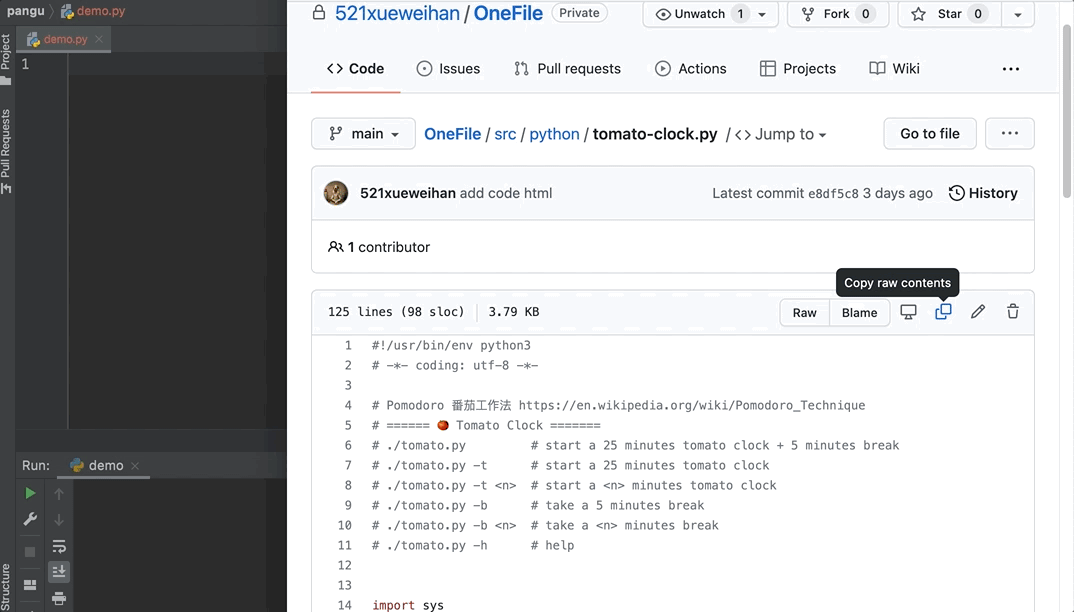### 3、迷你编译器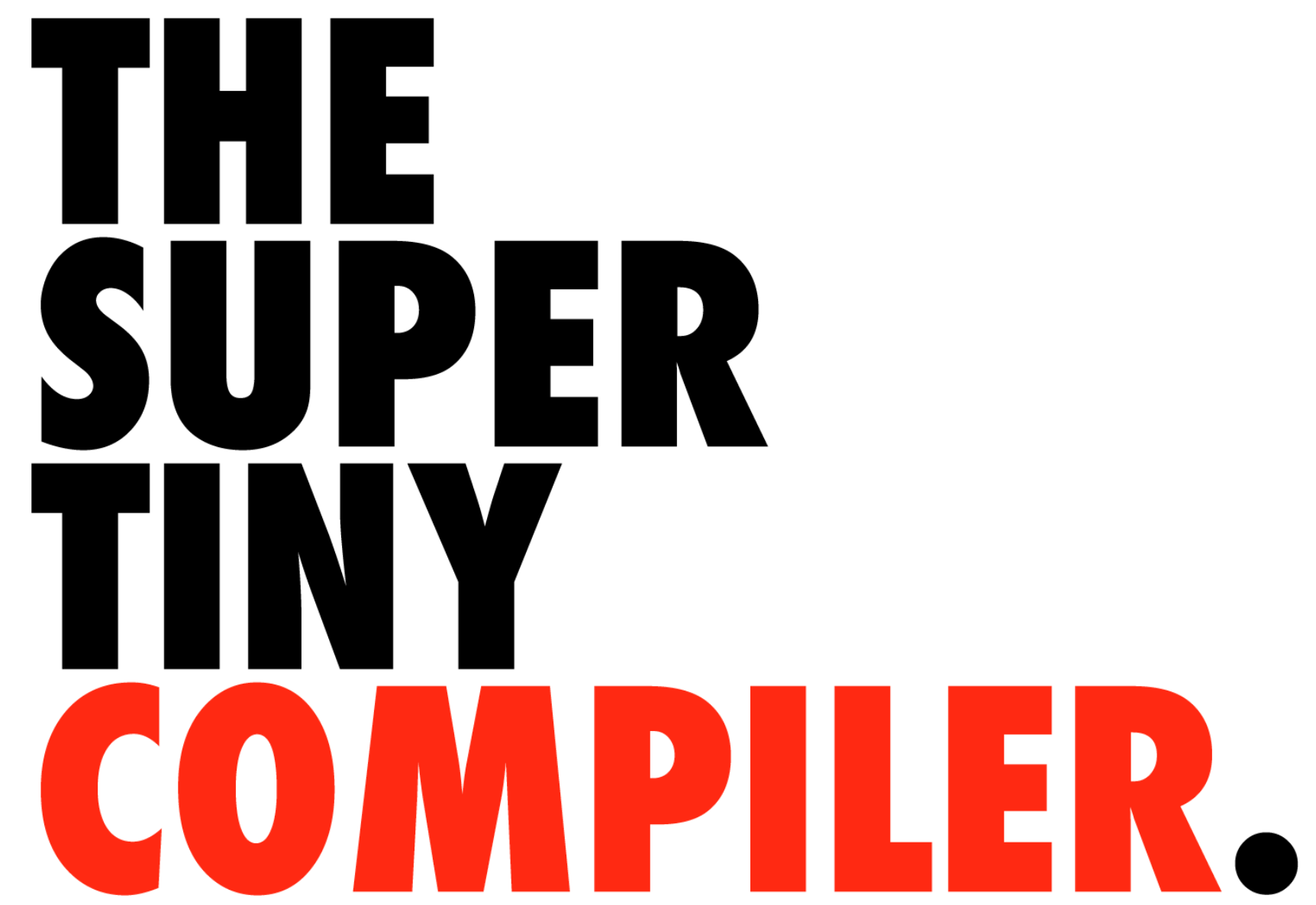`````` *                  LISP                      C
*
*   2 + 2          (add 2 2)                 add(2, 2)
*   4 - 2          (subtract 4 2)            subtract(4, 2)
*   2 + (4 - 2)    (add 2 (subtract 4 2))    add(2, subtract(4, 2))
``````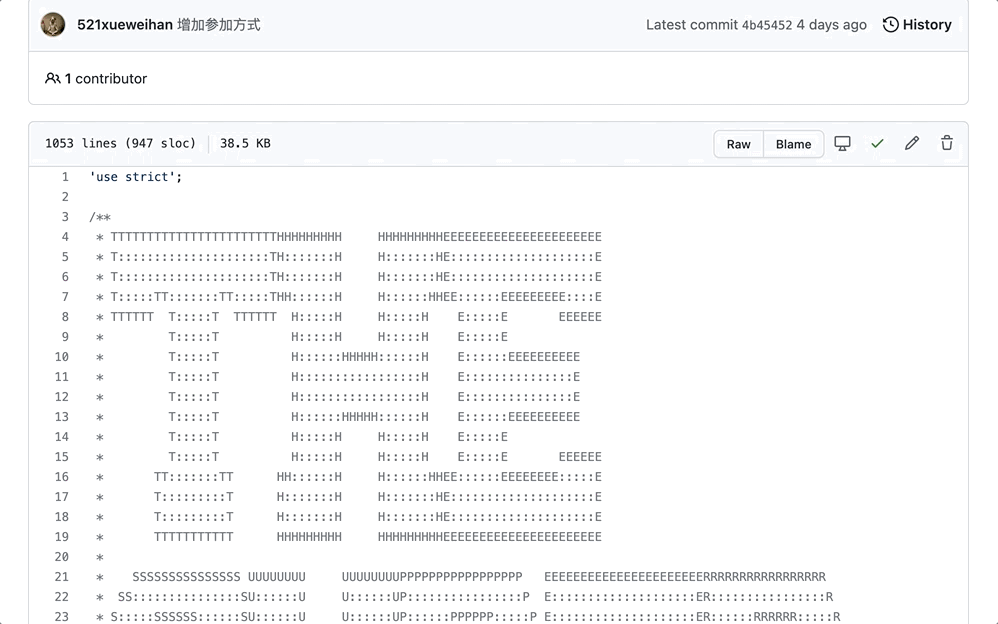### 4、字符视频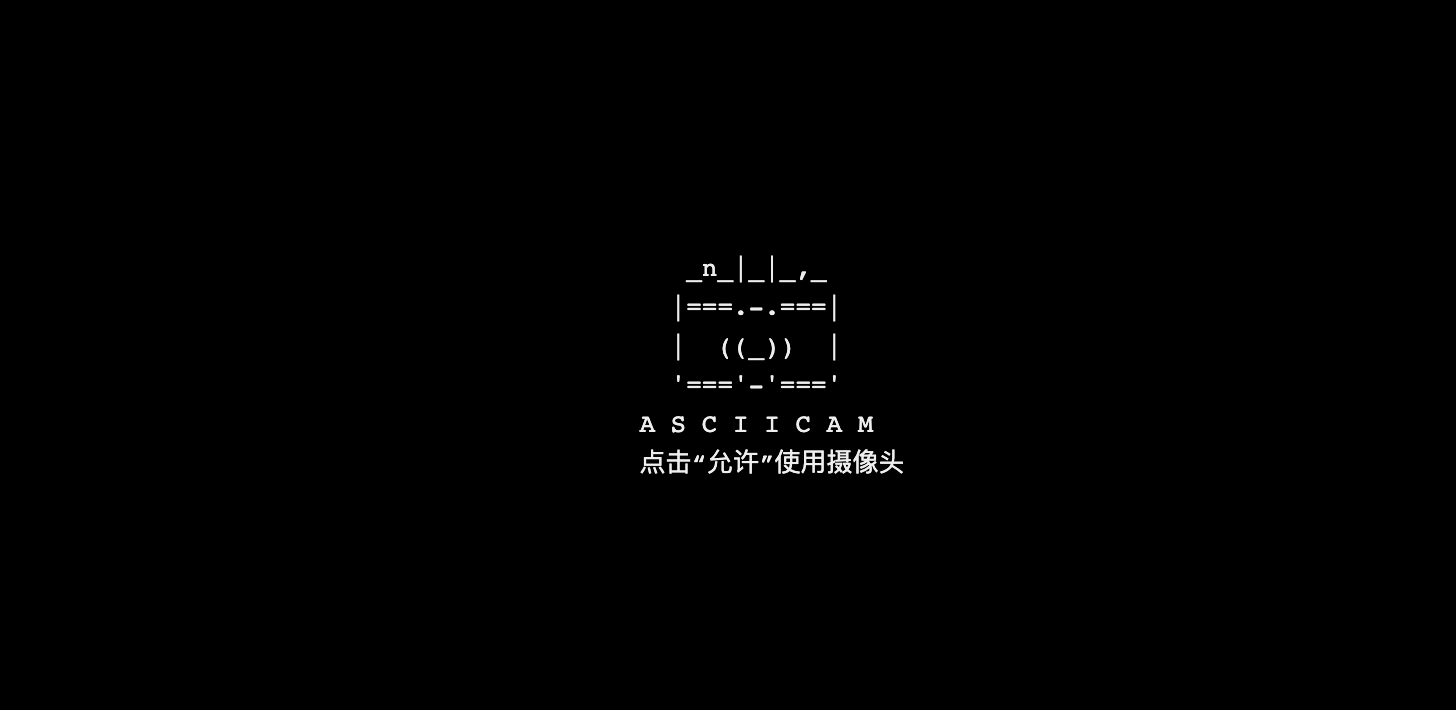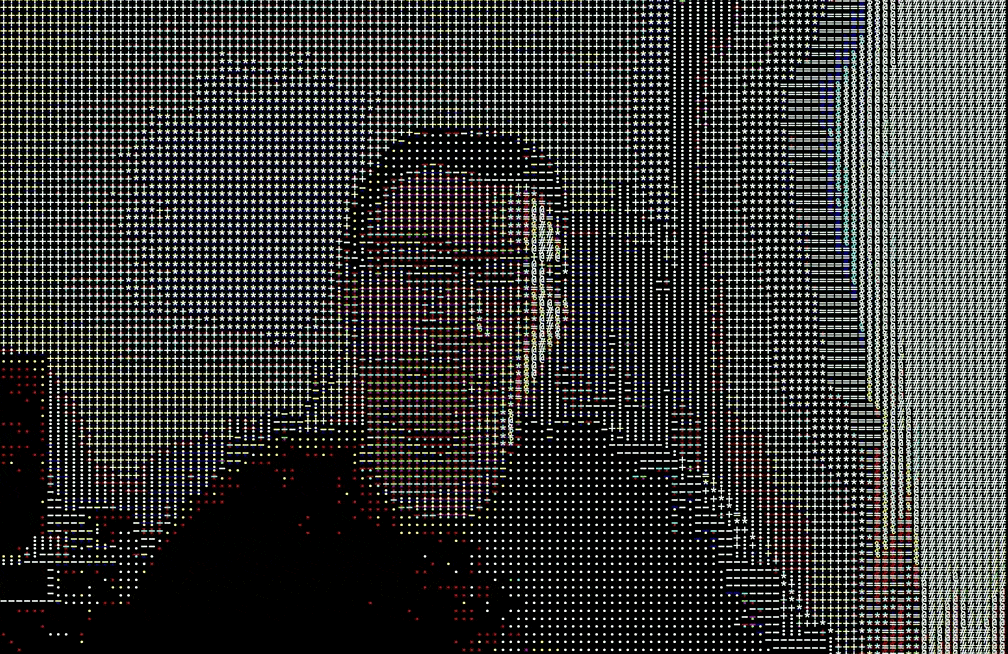### 5、太空侵略者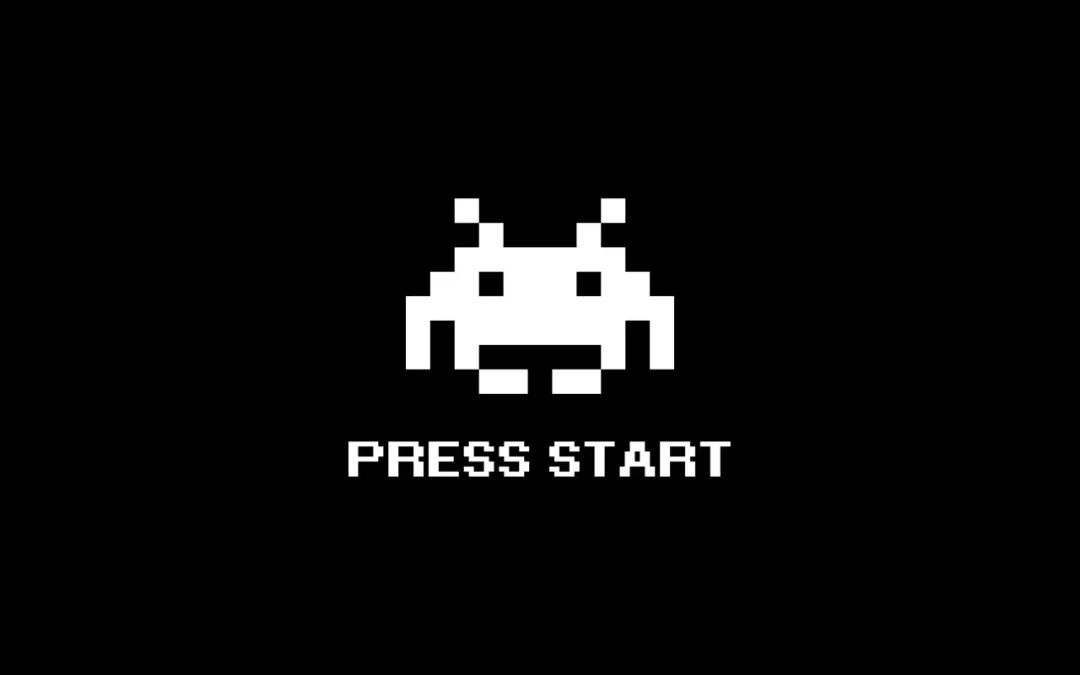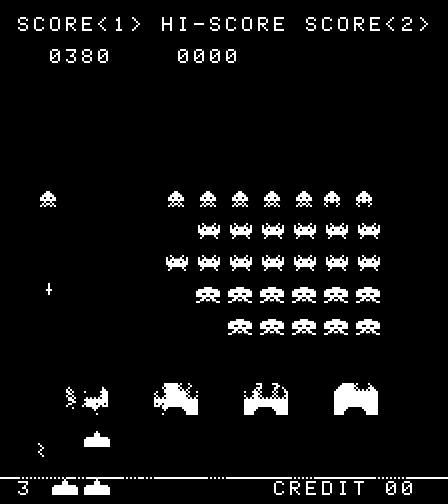## 开启开源之旅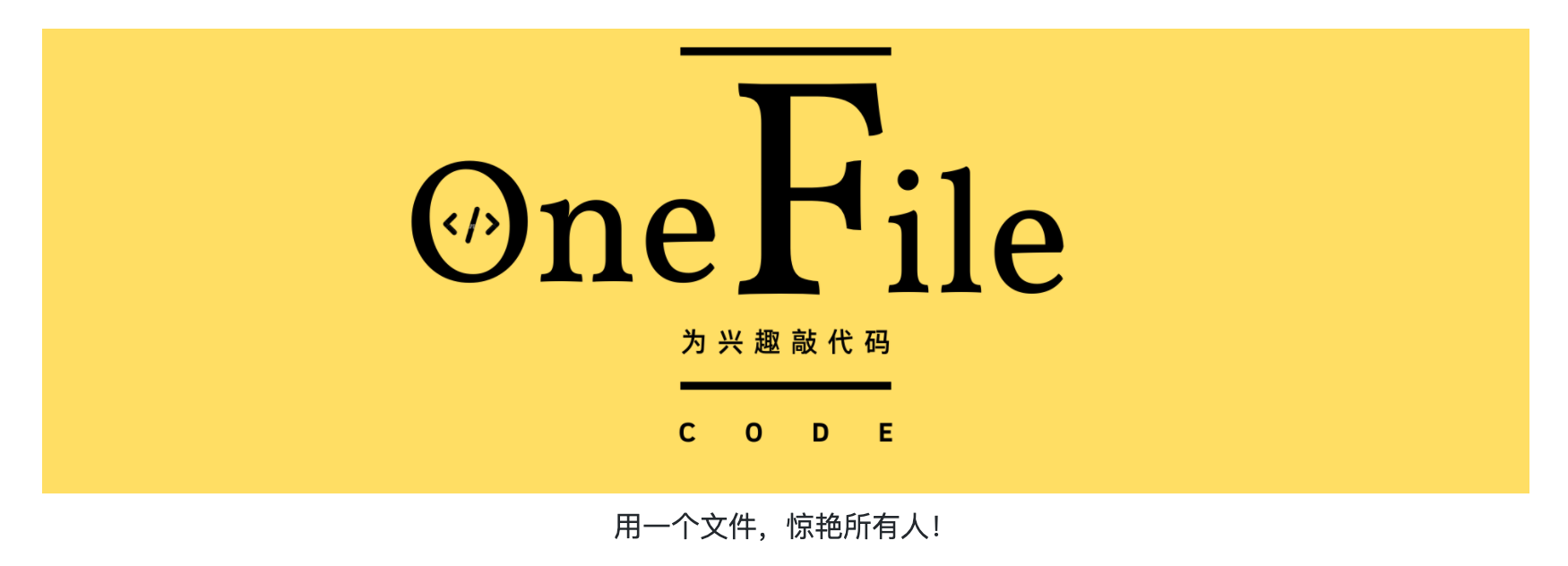• 单个文件、不可压缩、体积小于 1 MB
• 代码结构清晰，包含注释，行数小于 5000 行
• 不限编程语言，不可仅包含 Markdown 文件
• 不引入任何外部文件（图片、视频、CSS 等）
• 项目功能完整，具有学习或使用价值
• (可选)最多依赖一个开源库或框架